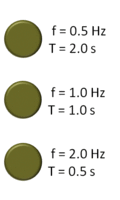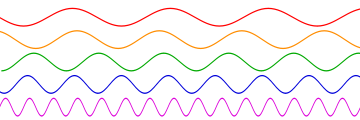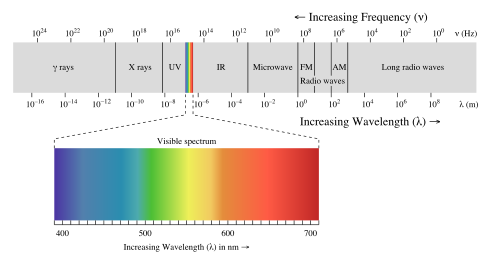# Frequency

﻿
FrequencyThree cyclically flashing lights, from lowest frequency (top) to highest frequency (bottom). f is the frequency in hertz (Hz), meaning the number of cycles per second. T is the period in seconds (s), meaning the number of seconds per cycle. T and f are reciprocals.

Frequency is the number of occurrences of a repeating event per unit time. It is also referred to as temporal frequency. The period is the duration of one cycle in a repeating event, so the period is the reciprocal of the frequency. For example, if a newborn baby's heart beats at a frequency of 120 times a minute, its period (the interval between beats) is half a second.

## Definitions and units

For cyclical processes, such as rotation, oscillations, or waves, frequency is defined as a number of cycles per unit time. In physics and engineering disciplines, such as optics, acoustics, and radio, frequency is usually denoted by a Latin letter f or by a Greek letter ν (nu).

In SI units, the unit of frequency is the hertz (Hz), named after the German physicist Heinrich Hertz: 1 Hz means that an event repeats once per second. A previous name for this unit was cycles per second.

A traditional unit of measure used with rotating mechanical devices is revolutions per minute, abbreviated RPM. 60 RPM equals one hertz.

The period, usually denoted by T, is the length of time taken by one cycle, and is the reciprocal of the frequency f:$T = \frac{1}{f}$

The SI unit for period is the second.

## MeasurementSinusoidal waves of various frequencies; the bottom waves have higher frequencies than those above. The horizontal axis represents time.

### By counting

Calculating the frequency of a repeating event is accomplished by counting the number of times that event occurs within a specific time period, then dividing the count by the length of the time period. For example, if 71 events occur within 15 seconds the frequency is:$f = \frac {71}{15 \,\mbox{sec}} \approx 4.7 \,\mbox{hertz} \,$

If the number of counts is not very large, it is more accurate to measure the time interval for a predetermined number of occurrences, rather than the number of occurrences within a specified time. The latter method introduces a random error into the count of between zero and one count, so on average half a count.

### By stroboscope

An older method of measuring the frequency of rotating or vibrating objects is to use a stroboscope. This is an intense repetitively flashing light (strobe light) whose frequency can be adjusted with a calibrated timing circuit. The strobe light is pointed at the rotating object and the frequency adjusted up and down. When the frequency of the strobe equals the frequency of the rotating or vibrating object, the object completes one cycle of oscillation and returns to its original position between the flashes of light, so when illuminated by the strobe the object appears stationary. Then the frequency can be read from the calibrated readout on the stroboscope. A downside of this method is that an object rotating at an integral multiple of the strobing frequency will also appear stationary.

### By frequency counter

Higher frequencies are usually measured with a frequency counter. This is an electronic instrument which measures the frequency of an applied repetitive electronic signal and displays the result in hertz on a digital display. It uses digital logic to count the number of cycles during a time interval established by a precision quartz time base. Cyclic processes that are not electrical in nature, such as the rotation rate of a shaft, mechanical vibrations, or sound waves, can be converted to a repetitive electronic signal by transducers and the signal applied to a frequency counter. Frequency counters can currently cover the range up to about 100 GHz. This represents the limit of direct counting methods; frequencies above this must be measured by indirect methods.

### Heterodyne methods

Above the range of frequency counters, frequencies of electromagnetic signals are often measured indirectly by means of heterodyning (frequency conversion). A reference signal of a known frequency near the unknown frequency is mixed with the unknown frequency in a nonlinear mixing device such as a diode. This creates a heterodyne or "beat" signal at the difference between the two frequencies. If the two signals are close together in frequency the heterodyne is low enough to be measured by a frequency counter. Of course, this process just measures the unknown frequency by its offset from the reference frequency, which must be determined by some other method. To reach higher frequencies, several stages of heterodyning can be used. Current research is extending this method to infrared and light frequencies (optical heterodyne detection).

## Frequency of waves

For periodic waves, frequency has an inverse relationship to the concept of wavelength; simply, frequency is inversely proportional to wavelength λ (lambda). The frequency f is equal to the phase velocity v of the wave divided by the wavelength λ of the wave:$f = \frac{v}{\lambda}.$

In the special case of electromagnetic waves moving through a vacuum, then v = c, where c is the speed of light in a vacuum, and this expression becomes:$f = \frac{c}{\lambda}.$

When waves from a monochrome source travel from one medium to another, their frequency remains exactly the same — only their wavelength and speed change.

## Examples

### Physics of light

Visible light is an electromagnetic wave, consisting of oscillating electric and magnetic fields traveling through space. The frequency of the wave determines its color: 4×1014 Hz is red light, 8×1014 Hz is violet light, and between these (in the range 4-8×1014 Hz) are all the other colors of the rainbow. An electromagnetic wave can have a frequency less than 4×1014 Hz, but it will be invisible to the human eye; such waves are called infrared (IR) radiation. At even lower frequency, the wave is called a microwave, and at still lower frequencies it is called a radio wave. Likewise, an electromagnetic wave can have a frequency higher than 8×1014 Hz, but it will be invisible to the human eye; such waves are called ultraviolet (UV) radiation. Even higher-frequency waves are called X-rays, and higher still are gamma rays.

All of these waves, from the lowest-frequency radio waves to the highest-frequency gamma rays, are fundamentally the same, and they are all called electromagnetic radiation. They all travel through a vacuum at the speed of light.

Another property of an electromagnetic wave is its wavelength. The wavelength is inversely proportional to the frequency, so an electromagnetic wave with a higher frequency has a shorter wavelength, and vice-versa.Complete spectrum of electromagnetic radiation with the visible portion highlighted

### Physics of sound

Sound is vibrations composed of frequencies able to be detected by ears. Frequency is the property of sound that most determines pitch.

Mechanical vibrations perceived as sound travel through all forms of matter: gases, liquids, solids, and plasmas. The matter that supports the sound is called the medium. Sound cannot travel through a vacuum.

The audible frequency range for humans is limited to frequencies between about 20 Hz and 20,000 Hz (20 kHz). Higher frequencies often become more difficult to hear with age. Other species have different hearing ranges. For example, some dog breeds can perceive vibrations up to 60,000 Hz.

### Line current

In Europe, Africa, Australia, Southern South America, most of Asia, and Russia, the frequency of the alternating current in household electrical outlets is 50 Hz (close to the tone G), whereas in North America and Northern South America, the frequency of the alternating current in household electrical outlets is 60 Hz (between the tones B♭ and B; that is, a minor third above the European frequency). The frequency of the 'hum' in an audio recording can show where the recording was made, in countries using a European, or an American, grid frequency.

## Period versus frequency

As a matter of convenience, longer and slower waves, such as ocean surface waves, tend to be described by wave period rather than frequency. Short and fast waves, like audio and radio, are usually described by their frequency instead of period. These commonly used conversions are listed below:

 Frequency Period (time) 1 mHz (10−3) 1 Hz (100) 1 kHz (103) 1 MHz (106) 1 GHz (109) 1 THz (1012) 1 ks (103) 1 s (100) 1 ms (10−3) 1 µs (10−6) 1 ns (10−9) 1 ps (10−12)

## Other types of frequency$\omega=2\pi f.\,$
In other words,$\sin(\omega t)=\sin(2\pi f t).\,$
• Spatial frequency is analogous to temporal frequency, but the time axis is replaced by one or more spatial displacement axes.
• Wavenumber is the spatial analogue of angular frequency. In case of more than one spatial dimension, wavenumber is a vector quantity.

## Frequency ranges

The frequency range of a system is the range over which it is considered to provide a useful level of signal with acceptable distortion characteristics. A listing of the upper and lower limits of frequency limits for a system is not useful without a criterion for what the range represents.

Many systems are characterized by the range of frequencies to which they respond. Musical instruments produce different ranges of notes within the hearing range. The electromagnetic spectrum can be divided into many different ranges such as visible light, infrared or ultraviolet radiation, radio waves, X-rays and so on, and each of these ranges can in turn be divided into smaller ranges. A radio communications signal must occupy a range of frequencies carrying most of its energy, called its bandwidth. Allocation of radio frequency ranges to different uses is a major function of radio spectrum allocation.

 Periodicity (disambiguation) Piano key frequencies Pink noise Pitch (music) Preselector Power bandwidth Range (music) Radar signal characteristics Radio window Rate (mathematics) Scientific pitch notation Signaling (telecommunications) Spectral width Spread spectrum Spectral component Spectrum allocation Symbol rate Transition band Transverter Ultrasound Upsampling Wavelength Whistle register Wideband audio

Wikimedia Foundation. 2010.

Synonyms:

### Look at other dictionaries:

• frequency — UK US /ˈfriːkwənsi/ noun [C or U] ► the number of times something happens within a particular period, or the fact of something happening often or a large number of times: »Once a week is a good frequency to back up all the data. the frequency of… …   Financial and business terms

• Frequency — Título Desafío al tiempo (Hispanoamérica) Frequency (España) Ficha técnica Dirección Gregory Hoblit Producción Gregor …   Wikipedia Español

• frequency — of vibration is the number of periods occurring in unit time. excitation frequency exciting frequency natural frequency resonant frequency …   Mechanics glossary

• Frequency — Fre quen*cy, n.; pl. {Frequencies}. [L. frequentia numerous attendance, multitude: cf. F. fr[ e]quence. See {Frequent}.] 1. The condition of returning frequently; occurrence often repeated; common occurence; as, the frequency of crimes; the… …   The Collaborative International Dictionary of English

• Frequency — (engl. für Frequenz) bezeichnet ein Musikfestival in Österreich, siehe Frequency (Musikfestival) einen Science Fiction Film aus dem Jahr 2000, siehe Frequency (Film) eine schwedische Metal Band Diese …   Deutsch Wikipedia

• Frequency — Éditeur SCE Développeur Harmonix Date de sortie 20 novembre 2001 …   Wikipédia en Français

• frequency — 1640s, fact of occurring often, from L. frequentia a crowding, crowd, from frequentem (see FREQUENT (Cf. frequent)). Earlier it had been used in a now obsolete sense of state of being crowded (mid 16c.); sense in physics, rate of recurrence,… …   Etymology dictionary

• frequency — [frē′kwənsfrē′kwən sē] n. pl. frequencies [ME < Fr < L frequentia < frequens: see FREQUENT] 1. Obs. a) the condition of being crowded b) a crowd 2. the fact of occurring often or repeatedly; frequent occurrence 3 …   English World dictionary

• frequency — frequency. См. частота мутаций. (Источник: «Англо русский толковый словарь генетических терминов». Арефьев В.А., Лисовенко Л.А., Москва: Изд во ВНИРО, 1995 г.) …   Молекулярная биология и генетика. Толковый словарь.

• frequency — frequency. См. частота рекомбинаций. (Источник: «Англо русский толковый словарь генетических терминов». Арефьев В.А., Лисовенко Л.А., Москва: Изд во ВНИРО, 1995 г.) …   Молекулярная биология и генетика. Толковый словарь.

• frequency — noun common occurrence, commonness, continuity, crebritas, cycle, frequence, frequentia, periodicity, prevalence, rate, recurrence, regularity, regularity of recurrence, repetition, repetitiveness, succession, usualness Burton s Legal Thesaurus.… …   Law dictionary